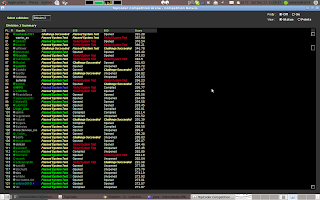## Saturday, December 17, 2011

### SRM527:

Level 1 Level 2 Level 3
Div 1 Level 1 Level 2 Level 3
Div 2 Level 1 Level 2 Level 3

## Tutorials:SRM527

### Solution

It's the same with Div2-Level2.

### Solution

Greedy + brute forces.
1st, convert the coins_sum to binary number.
2nd, if the sum is greater than the N-length binary number could represent, use the first coin (or the one with greatest value) to represent the part which is greater than the one could represent.
3rd, count the number of coins used by now.
4th, use the ones with greatest value to increase the number of coins could be used by split it into 2 coins with its half value. This step is implemented by Greedy.

Above all, I used brute forces here.

### Source Code:

/**
* @author antonio081014
* @since Dec 17, 2011, 9:51:30 AM
*/

public class P8XCoinChangeAnother {

public long[] solve(int N, long coins_sum, long coins_count) {
String sum = Long.toBinaryString(coins_sum);
int len = sum.length();
long[] ret = new long[N];
long cnt = 0;
while (len > N) {
cnt = cnt * 2 + (sum.charAt(0) - '0');
sum = sum.substring(1);
len--;
}
long current = 0;
for (int i = 0; i < N; i++) {
// current += sum.charAt(len - 1 - i) - '0';
ret[i] = sum.charAt(len - i - 1) - '0';
}
ret[N - 1] += 2 * cnt;
// current += 2 * cnt;

for (int i = 0; i < N; i++) {
current += ret[i];
System.out.print(ret[i] + " ");
}
System.out.println(current);
int index = N - 1;
while (coins_count > current && index > 0) {
long tmp = coins_count - current;
if (tmp > ret[index]) {
ret[index - 1] += 2 * ret[index];
current += ret[index];
ret[index] = 0;
index--;
} else {
ret[index - 1] += 2 * tmp;
current += tmp;
ret[index] -= tmp;
break;
}
}

if (current != coins_count) {
ret = new long;
}

return ret;
}

// <%:testing-code%>
}

### Solution

This one could be solved by dynamic programming.
The code will be attached later.

### Source Code:

/**
*
* dp[i][j] represents the maximum score with i nodes and j max-degrees.
*
* Then, we add one node with k-degree, we could get:
* dp[i+1][j+k] = Math.max(dp[i+1][j+k], score[k-1] + dp[i][j]);
*
* At last, we only need to return the maximum value with
* N+1 (since N is the number of available degrees, which is nodes number - 1) nodes,
* and 2*N degree.
* */

/**
* @author antonio081014
* @since Dec 29, 2011, 9:54:00 AM
*/

public class P8XGraphBuilder {

public int[][] dp;

public int solve(int[] scores) {
int N = scores.length;
dp = new int[N + 2][2 * N + 2];
for (int i = 0; i < N + 2; i++)
for (int j = 0; j < 2 * N + 2; j++)
dp[i][j] = Integer.MIN_VALUE;
dp = 0;
for (int i = 0; i <= N; i++) {
for (int j = 0; j <= 2 * N; j++) {
if (dp[i][j] == Integer.MIN_VALUE)
continue;
for (int k = 1; k <= N && k + j <= 2 * N; k++) {
dp[i + 1][j + k] = Math.max(dp[i + 1][j + k], scores[k - 1]
+ dp[i][j]);
}
}
}
return dp[N + 1][2 * N];
}
// <%:testing-code%>
}

### Solution

Totally brute force.

### Source Code:

/**
* @author antonio081014
* @since Dec 17, 2011, 9:02:35 AM
*/

public class P8XMatrixTransformation {
public String solve(String[] original, String[] target) {
return counter(original) == counter(target) ? "YES" : "NO";
}

public int counter(String[] matrix) {
int ret = 0;
for (int i = 0; i < matrix.length; i++) {
for (int j = 0; j < matrix[i].length(); j++) {
if (matrix[i].charAt(j) == '1')
ret++;
}
}
return ret;
}

// <%:testing-code%>
}